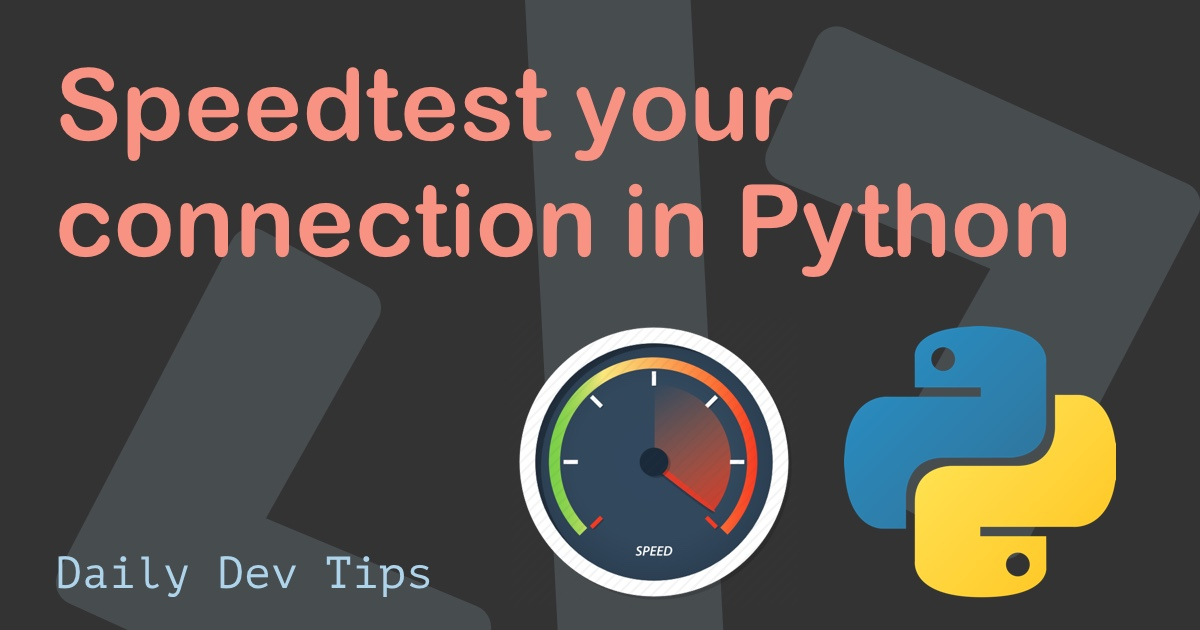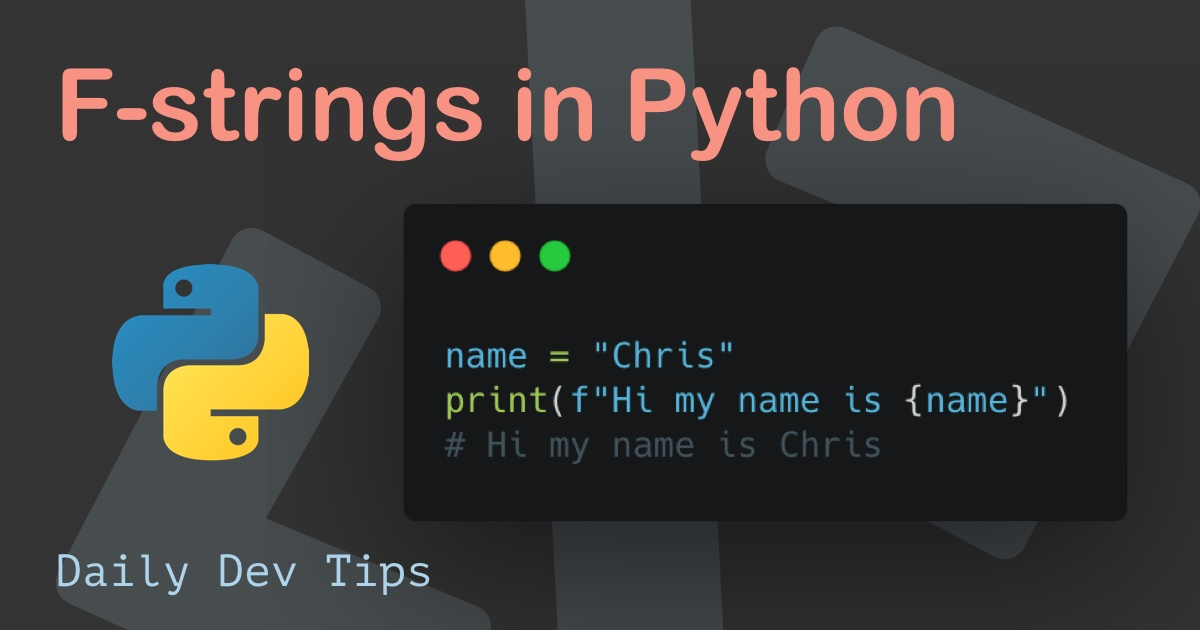Subscribe

# NumPy arrays, a broader look

✍️

A deeper look into arrays in NumPy (Python)

3 Jun, 2021 · 4 min read

Now that we installed NumPy, let's have a look at some of the details of what we can do with it.

For today's article, we'll be at the following elements of NumPy arrays.

• Dimensions
• Joining arrays
• Splitting arrays

## Dimensions in NumPy arrays permalink

When it comes to NumPy arrays, we can talk about several dimensions for this array. I'll be walking through some of these dimensions so you can see the difference and meaning between them.

0-D Arrays Talking about the first level, a 0-D, we mean each value in an array! So to write it, this would be a 0-D array.

``````arr = np.array("0-D")
print(arr)
# '0-D'``````

1-D Arrays

When this array has multiple elements, we call it a 1-D array. So, in general, a 1-Dimensional array contains multiple 0-D elements.

``````arr = np.array(["1-D", "1-D"])
print(arr)
# ['1-D' '1-D']``````

2-D Arrays

As you can imagine, a 2-D array is a combination of multiple 1-D arrays.

``````arr = np.array([["2-D", "2-D"], ["2-D", "2-D"]])
print(arr)
# [['2-D' '2-D'] ['2-D' '2-D']]``````

3-D Arrays

And again, if we have multiple 2-D arrays inside a bigger one, we can call it a 3-D array like this.

``````arr = np.array([[[3, 3], [3, 3]], [[3, 3], [3, 3]]])
print(arr)
# [[[3, 3], [3, 3]], [[3, 3], [3, 3]]]``````

If you are unsure about the dimensions and how many you got, you can use the `ndim` attribute to retrieve this.

``````a = np.array(0)
b = np.array([[2, 2], [2, 2]])
c = np.array([[[3, 3], [3, 3]], [[3, 3], [3, 3]]])
print(a.ndim)
# 0
print(b.ndim)
# 2
print(c.ndim)
# 3``````

## Joining arrays with NumPy permalink

Sometimes we need to join multiple arrays, and NumPy has the `concatenate` function for this.

Let's say we have two 1-D arrays we want to join into one bigger one.

``````arr1 = np.array([1, 2, 3])
arr2 = np.array([4, 5, 6])
arr = np.concatenate((arr1, arr2))
print(arr)
# [1 2 3 4 5 6]``````

We can also combine a further dimension array.

``````arr1 = np.array([[1, 2], [3, 4]])
arr2 = np.array([[5, 6], [7, 8]])
arr = np.concatenate((arr1, arr2))
print(arr)
# [[1 2] [3 4] [5 6] [7 8]]``````

But in some cases, we might want to combine the 1,2, and 5,6 since they are on the same dimension.

For this, we can specify the axis attribute on the concatenate.

``````arr = np.concatenate((arr1, arr2), axis=1)
# [[1 2 5 6] [3 4 7 8]]``````

When combining arrays, we can also use `stacking`; it's the same but always done along a new axis.

``````arr1 = np.array([1, 2, 3])
arr2 = np.array([4, 5, 6])
arr = np.stack((arr1, arr2), axis=1)
print(arr)
# [[1 4] [2 5] [3 6]]``````

You see, this combines the 1,4 and 2,5 etc.?

We can also choose to stack along rows using `hstack`.

``````arr1 = np.array([1, 2, 3])
arr2 = np.array([4, 5, 6])
arr = np.hstack((arr1, arr2))
print(arr)
# [1 2 3 4 5 6]``````

Now it's one array again.

Another option is to stack based on columns using the `vstack` function.

``````arr1 = np.array([1, 2, 3])
arr2 = np.array([4, 5, 6])
arr = np.vstack((arr1, arr2))
print(arr)
# [[1 2 3] [4 5 6]]``````

The last option is to use the `hstack` along the height (depth) of the array.

``````arr1 = np.array([1, 2, 3])
arr2 = np.array([4, 5, 6])
arr = np.dstack((arr1, arr2))
print(arr)
# [[[1 4] [2 5] [3 6]]]``````

So all and all, we have the option to make about every array dimension when combining arrays.

## Splitting arrays with NumPy permalink

After looking at combining, let's look at how we can split arrays.

For this, we use the `array_split` method.

The super cool feature to me is that you can state how many splits you want! So let's say we want to split this array into three pieces.

``````arr = np.array([1, 2, 3, 4, 5, 6])
split = np.array_split(arr, 3)
print(split)
# [[1,2], [3,4], [5,6]]``````

This is one feature that is super handy when handling large data sets. This also doesn't need equal items. It will make a smaller want in the end.

Of course the splitting also works with multi-level arrays.

Let's try and split this 3-D array into two pieces.

``````arr = np.array([[[3, 3], [3, 3]], [[3, 3], [3, 3]]])
split = np.array_split(arr, 2)
print(split)
# [[[[3, 3], [3, 3]]], [[[3, 3], [3, 3]]]]``````

And much like the concatenate, we can split on a specific axis with the axis property.

And like the `stack` option split also comes with:

• `hsplit`: Split along rows
• `vsplit`: Split along columns
• `dsplit`: Split along height (depth)

And with that, I just want to tell you again how powerful these arrays in NumPy are! If you ever had to split an array in JavaScript, you will have noted how much manual work it can be.

Tweet this tip

### Speedtest your connection in Python

21 Jun, 2021 · 2 min read### F-strings in Python

7 Jun, 2021 · 2 min readJoin 2101 devs and subscribe to my newsletter

• 1000 articles written
• 2101 devs subscribed
• 529116 words written